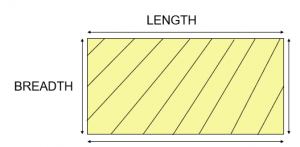# Area of Rectangle

Area of Rectangle is equal to the product of its length and breadth.

Area of Rectangle = Length x BreadthQuestion 1

Find the area of a rectangle in which:
length = 5 cm and breadth = 8 cm

Explanation:

Given,
length = 5 cm
Area of a rectangle = ( length x breadth ) cm²

= ( 5 x 8 ) cm²

= 40 cm²

Question 2

Find the area of a rectangle in which:
length = 11 cm and breadth = 6 cm

Explanation:

Given,
length = 11 cm
Area of a rectangle = ( length x breadth ) cm²

= ( 11 x 6 ) cm²

= 66 cm²

Question 3

Find the area of rectangle in which:
length = 18 cm and breadth = 15 cm

Explanation:

Given,
length = 18 cm
Area of rectangle = ( length x breadth ) cm²

= ( 18 x 15 ) cm²

= 270 cm²

Question 4

Find the area of rectangle in which: length = 25 cm and breadth = 12 cm

Explanation:

Given,
length = 25 cm

Area of rectangle = ( length x breadth ) cm²

= ( 25 x 12 ) cm²

= 300 cm²

Question 5

Find the area of rectangle in which:
length = 21 cm and breadth = 9 cm

Explanation:

Given,
length = 21 cm

Area of rectangle = ( length x breadth ) cm²

= ( 21 x 9 ) cm²

= 189 cm²

Question 6

Find the area of rectangle in which:
length = 15 cm and breadth = 12 cm

Explanation:

Given,
length = 15 cm